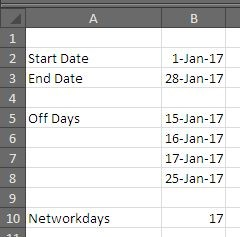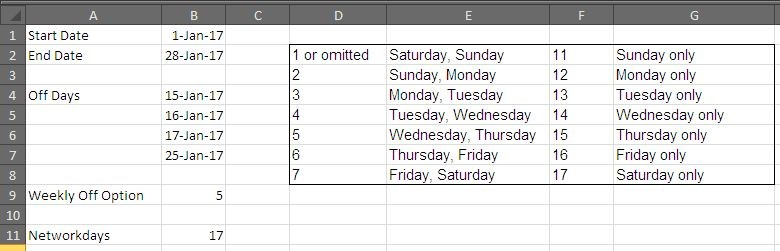# Calculating Workdays With Different MethodsNetworking days are important for both an employee and the employer. In todays post we will see how NETWORKDAYS can be calculated in Excel. MS Excel has some built in formulas to calculate network days.

In Excel 2010, we have following formulas available for calculating networking days.

• NETWORKDAYS

• NETWORKDAYS.INTL

Both these formulas have a little different syntax. We will take them up one by one and see how they can be used to find networking days.

The NETWORKDAYS() Formula:

The NETWORKDAYS() takes three arguments to calculate the working days i.e. a start date, an end date and an optional argument that consists of holidays, if you want them to be subtracted from the working days.

The Syntax is like following:

NETWORKDAYS(start_date, end_date, [holidays])

The NETWORKDAYS.INTL() Formula:

The NETWORKINGDAYS.INTL() takes into account the fact that across the world, weekend days varies i.e. week start on differ days and ends of different days. This is also true for workers that have rotational week according to their job requirement. Besides there is also a holiday’s optional argument that can accommodate the exceptional holidays.

The Syntax is like following:

NETWORKDAYS(start_date, end_date, weekend, [holidays])

These two function combined can be really helpful specially for Human Resource and Payroll management purposes.

Lets see how they work with following examples:

The NETWORKDAYS() Example:

Consider a situation where a Payroll manager is interested in finding one of his employees total working days. He knows that the employee has availed medical leaves between 15 Jan 2017 to 17th 2017 and on 25th Jan 2017.

When calculating workdays between 1st Jan to 28th Jan, the following sheet could assist him in this task.The following formula yields the displayed result: =NETWORKDAYS(B2,B3,B5:B8)

Here B2 is the start date, B3 is the end date, the range B5:B8 describes the medical leave availed by the employee. The total days thus worked by the employees in Jan 2017 are 17 days – (excluding Saturday and Sundays).

The NETWORKDAYS.INTL() Example

Now in order to elaborate the formula, add another criteria to the situation described above, that the Worker has weekly off on Wednesday and Thursday. The modified sheet is displayed in the following picture.Surprisingly, the formula gave us 17 as result.

The formula that yields us this result is following:

=NETWORKDAYS.INTL(B1,B2,B9,B4:B7)

In this formula, B1 and B2 are start and end dates respectively; B9 is the option that let us set the weekend for the employee – i.e. Wednesday and Thursday. And B4:B7 is the list of vacations taken by the employee on medical reasons.

So these were the two cases where can use excel to calculate networking days. Hope you have like the post. We will revert with more useful posts soon. Thank you.